# What is Determinants

## What is Determinants

• A determinant is a scalar value associated with a square matrix.
• It serves as a useful tool in solving systems of linear equations and finding the inverse of matrices.
• The determinant of a square matrix $[A_{ij}]$ is given |A|
• iF $A=\begin{bmatrix} 1 & 2 \\ 3 & -1 \\ \end{bmatrix}$
then Determinant is given by
$|A|=\begin{vmatrix} 1 & 2 \\ 3 & -1 \\ \end{vmatrix}$

## Determinant of a 2x2 Matrix

For a 2x2 matrix $A$ given by
$A = \begin{vmatrix} a & b \\ c & d \end{vmatrix}$

The determinant $|A|$ is calculated as:
$|A| = ad - bc$

## Determinant of a 3x3 Matrix

For a 3x3 matrix $B$ given by

$B = \begin{bmatrix} a & b & c \\ d & e & f \\ g & h & i \end{bmatrix}$

Determinant of a matrix of order three can be determined by expressing it in terms of second order determinants. This is known as expansion of a determinant along a row (or a column). There are six ways of expanding a determinant of order 3 corresponding to each of three rows (R1, R2 and R3 ) and three columns (C1, C2 and C3) giving the same value

Expansion Along R1
The determinant $|B|$ is calculated as
$|B| = (-1)^{1 +1} a(ei - fh) + (-1)^{1 +1} b(di - fg) + (-1)^{1 +3}c(dh - eg)$

This is obtained by
1. Multiply first element a of R1 by $(–1)^{1 + 1} [(–1)^{\text{sum of suffixes in a}}]$ and with the second order determinant obtained by deleting the elements of first row (R1) and first column (C1 ) of | A | as a lies in R1 and C1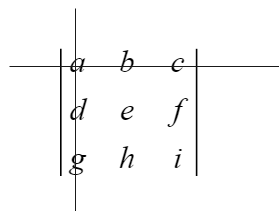$(-1)^{1 + 1} a \begin{vmatrix} e & f \\ h & i \\ \end{vmatrix}$
$=(-1)^{1 +1} a(ei - fh)$
2.Multiply 2nd element b of R1 by $(–1)^{1 + 2} [(–1)^{\text{sum of suffixes in b}}]$ and the second order determinant obtained by deleting elements of first row (R1) and 2nd column (C2) of | A | as b lies in R1 and C2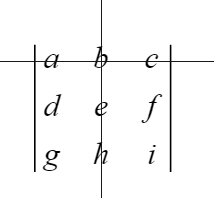$(-1)^{1 + 2} b \begin{vmatrix} d & f \\ g & i \\ \end{vmatrix}$
$=(-1)^{1 +2} b(di - fg)$
3. Multiply third element c of R1 by $(–1)^{1 + 3} [(–1)^{\text{sum of suffixes in c}}]$ and the second order determinant obtained by deleting elements of first row (R1) and third column (C3) of | A | as c lies in R1 and C3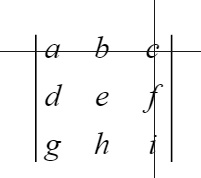$(-1)^{1 + 3} c \begin{vmatrix} d & e \\ g & h \\ \end{vmatrix}$
$=(-1)^{1 +3} c(dh - eg)$

Therefore
|B|=aei -afh -bdi + bfg + cdh -ceg

Expansion Along R2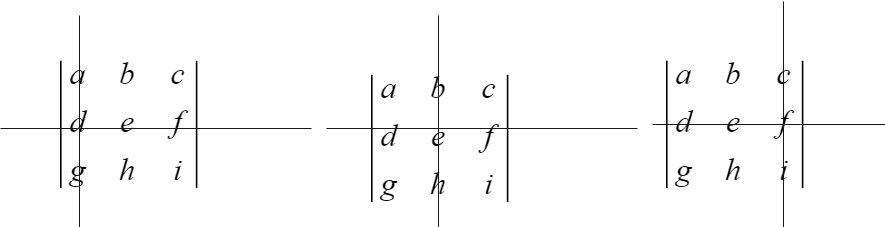we can write as
$|B| = (-1)^{2 +1} d(bi - ch) + (-1)^{2 +2} e(ai - cg) + (-1)^{2 + 3} f(ah - bg)=-bdi+ cdh + aei -acg -fah +fbg=aei -afh -bdi + bfg + cdh -ceg$
So it is the same value

Expansion Along R3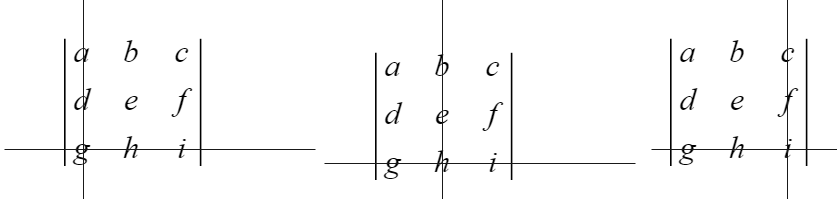we can write as
$|B| = (-1)^{3 +1} g(bf - ec) + (-1)^{3 +2} h(af - cd) + (-1)^{3 + 3} i(ae - bd)=bgf - gec - haf +hcd +aei -bdi=aei -afh -bdi + bfg + cdh -ceg$
So it is the same value

Expansion Along C1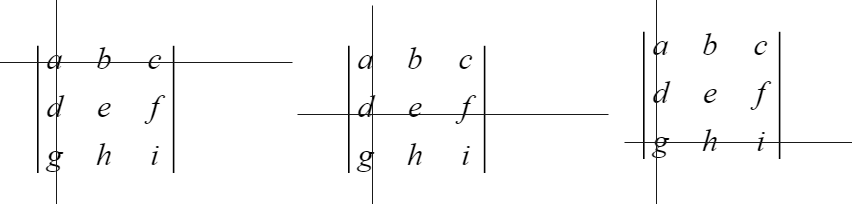we can write as
$|B| = (-1)^{1 +1} a(ei - fh) + (-1)^{2 +1} d(bi - ch) + (-1)^{3 + 1} g(bf - ec)=aei - afh - bdi +hcd +gbf -gec=aei -afh -bdi + bfg + cdh -ceg$
So it is the same value

Expansion Along C2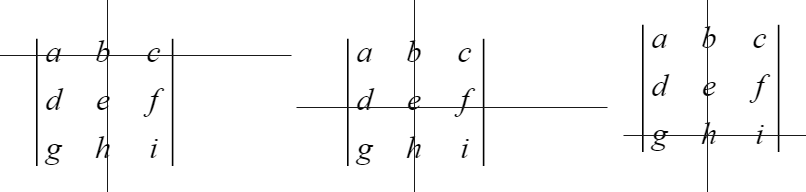we can write as
$|B| = (-1)^{1+ 2} b(di - fg) + (-1)^{2 +2} e(ai - cg) + (-1)^{3 + 2} h(af - cd)=-bdi + bfg +aei -ecg -haf +hcd=aei -afh -bdi + bfg + cdh -ceg$
So it is the same value

Expansion Along C2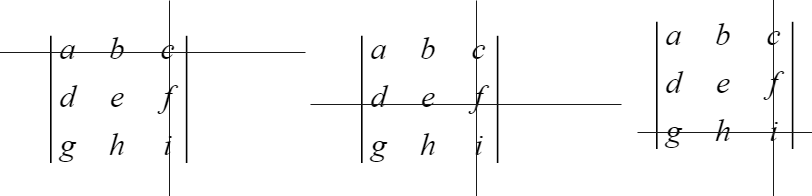we can write as
$|B| = (-1)^{1 +3} c(dh - eg) + (-1)^{2 +3} f(ah - bg) + (-1)^{3 + 3} i(ae - bd)=cdh ceg -afh +bfg +aei -bdi=aei -afh -bdi + bfg + cdh -ceg$
So it is the same value

• Notes NCERT Solutions & Assignments

Go back to Class 12 Main Page using below links